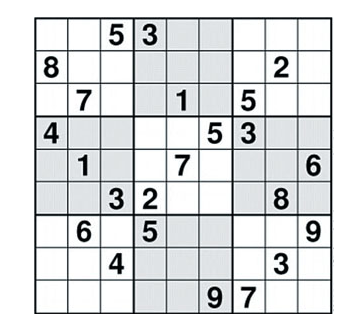# 数独游戏-蓝桥杯-C语言005300000
800000020
070010500
400005300
010070006
003200080
060500009
004000030
000009700

145327698
839654127
672918543
496185372
218473956
753296481
367542819
984761235
521839764

800000000
003600000
070090200
050007000
000045700
000100030
001000068
008500010
090000400

812753649
943682175
675491283
154237896
369845721
287169534
521974368
438526917
796318452

CPU消耗  < 2000ms

#include<stdio.h>

//读取未完成的数独矩阵

void readMatrix(int array){

int row, //行

col; //列

for(row = 0;row < 9;row++){

for(col = 0;col < 9;col++)

scanf(" %1d",&array[row][col]); /****注意%1d及前面是有空格的****/

getchar();

}

}

//判断在行和列上有没有重复数字（不重复true,重复false）

bool isRowAndColRepeat(int array,int row,int col,int num){

int i; //计数

//判断行上有没有重复的数字

for(i = 0;i < 9;i++) if(array[row][i] == num) return false;

//判断列上有没有重复的数字

for(i = 0;i < 9;i++) if(array[i][col] == num) return false; return true; }

//判断 判断位置 属于哪个3x3九宫格

int getRowOrCol(int num){ if(num < 3) return 0; else if(num < 6) return 3; else return 6; }

//判断3x3九宫格（即同色九宫格）内是否有重复的数字（不重复true,重复false）

bool isBlockRepeat(int array,int row,int col,int num){

int getRowOrCol(int num); //得到3x3九宫格起始位置的函数原型声明

int rowStart, //行号

colStart, //列号

i,j; //计数

rowStart = getRowOrCol(row);

colStart = getRowOrCol(col);

for(i = rowStart;i < rowStart+3;i++)

for(j = colStart;j < colStart+3;j++)

if(array[i][j] == num) return false; return true;

}

//对数独九宫格进行深度优先检索

void dfs(int array,int row,int col){

int i,j; //计数 //递归，设置出口：如果行号row超出数组行下标，则退出递归即输出答案

if(row > 8){

for(i = 0;i < 9;i++){ for(j = 0;j < 9;j++) printf("%d",array[i][j]); printf("\n"); }

return; }

//如果array[row][col] == 0即该位置的数未确定（不是题目中给好的数，可以更改）

if(array[row][col] == 0){

//从1~9开始试数 for(i = 1;i < 10;i++) //如果1~9中有合适的数，则该位置的数为该数，并开始遍历下一个位置 if(isRowAndColRepeat(array,row,col,i) && isBlockRepeat(array,row,col,i)){

array[row][col] = i; dfs(array,row + (col+1)/9,(col+1)%9); //当每一行的单元格都遍历过，再进入下一行

}

array[row][col] = 0; //如果没有(不是)合适的数，则重置为0

}else{

// 如果该位置的数已确定则跳过该位置，遍历下一个位置 dfs(array,row + (col+1)/9,(col+1)%9);

//当每一行的单元格都遍历过，再进入下一行

}

}

int main()

{

int array; readMatrix(array); //读取数独题目 dfs(array,0,0);

//从第一个单元开始深度优先检索

return 0;

}